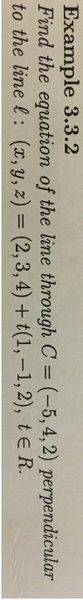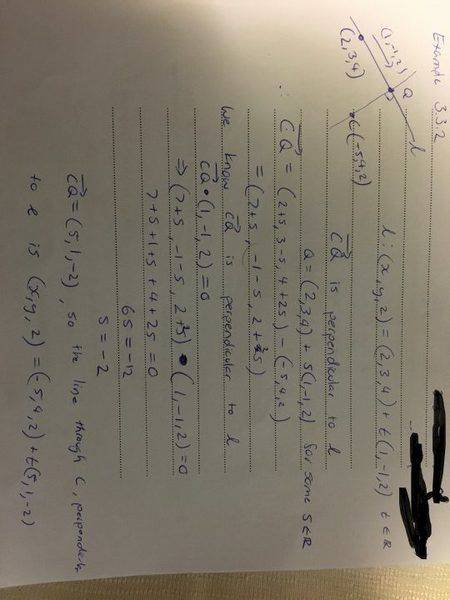# Vector equation perpendicular to two equations

• I
• 53Mark53

#### 53Mark53

How do I find the vector equation of the line which passes through (-3/2,-3/2,1/2) and is perpendicular to both x+1=y/3=-z and 2x+1=2y+1=z-5/2

I know how to do it using one equation but I am unsure about how to do it using two equations

Thanks

Find tangent vector to those lines and take their cross product. Then find the equation of a line with a tangent equal to that cross product which passes from that point.

Find tangent vector to those lines and take their cross product. Then find the equation of a line with a tangent equal to that cross product which passes from that point.
Could you please show an example I can't find anything about tangent vectors in my book

Thanks

For example for the line ##x+1=\frac y 3=-z##: At first we should transform it to the parametric form, simply write ## z=-t ##, then we'll have ## \frac y 3=t \Rightarrow y=3t ## and ## x=t-1 ##. So each point on the line has coordinates of the form ## (t-1,3t,-t) ## and the tangent vector to this line is simply the derivative of the latter expression w.r.t. t which is ## (1,3,-1) ##.

For example for the line ##x+1=\frac y 3=-z##: At first we should transform it to the parametric form, simply write ## z=-t ##, then we'll have ## \frac y 3=t \Rightarrow y=3t ## and ## x=t-1 ##. So each point on the line has coordinates of the form ## (t-1,3t,-t) ## and the tangent vector to this line is simply the derivative of the latter expression w.r.t. t which is ## (1,3,-1) ##.
My class has not learned anything about using derivatives with vectors is there another way to solve this without using derivatives?

thanks

My class has not learned anything about using derivatives with vectors is there another way to solve this without using derivatives?

thanks

What textbook are you using?
I think its better for me to take a look at it to see what tools you have at hand.

What textbook are you using?
I think its better for me to take a look at it to see what tools you have at hand.

This is the example we had from classCan you write the equation of those two lines in the form ## (x,y,z)=\vec a + \vec b t ##? This is the form in which the equation of the line is given in your example from the class.

Can you write the equation of those two lines in the form ## (x,y,z)=\vec a + \vec b t ##? This is the form in which the equation of the line is given in your example from the class.

(x,y,z)=(-1/2,-1/2-5/2)+t(1/2,1/2,1)
and
(x,y,z)=(-1,0,0)+s(1,3,-1)

would I have to combine these?

(x,y,z)=(-1/2,-1/2-5/2)+t(1/2,1/2,1)
and
(x,y,z)=(-1,0,0)+s(1,3,-1)

would I have to combine these?

Good. Now those constant vectors which are the coefficients of t and s are the tangent vectors to those lines. Now you can either take the vector product of those tangent vectors as the tangent vector for your line or consider a vector with unknown components and set its scalar product with both tangent vectors to zero and find the components and take it as the tangent vector for your line.

•53Mark53
Good. Now those constant vectors which are the coefficients of t and s are the tangent vectors to those lines. Now you can either take the vector product of those tangent vectors as the tangent vector for your line or consider a vector with unknown components and set its scalar product with both tangent vectors to zero and find the components and take it as the tangent vector for your line.
Thanks I got it now